## Model Engine Using n-D Adaptive Lookup Table

### Objectives

In this example, you learn how to capture the time-varying behavior of an engine using an n-D adaptive lookup table. You accomplish the following tasks using the Simulink® software:

• Simulate the model to update the lookup table values dynamically.

• Export the adapted lookup table values to the MATLAB® workspace.

• Lock a specific cell in the table during adaptation.

• Disable the adaptation process and use the adaptive lookup table as a static lookup table.

In this example, you use the data in `vedata.mat` which contains the following variables measured from an engine:

• `X` — 10 input breakpoints for intake manifold pressure in the range [10,100]

• `Y` — 36 input breakpoints for engine speed in the range [0,7000]

• `Z` — 10x36 matrix of table data for engine volumetric efficiency

The output volumetric efficiency of the engine is time varying, and a function of two inputs—intake manifold pressure and engine speed. The data in the MAT-file is used to generate the time-varying input and output (I/O) data for the engine.

In next section, you open a preconfigured Simulink model.

### Engine Data Model

`load vedata.mat`

Open the preconfigured model.

`open_system('enginetable1_data')`

### Building a Model Using Adaptive Lookup Table Blocks

In this portion of the tutorial, you learn how to build a model of an engine using an Adaptive Lookup Table block.

1. The Experimental Data subsystem in the Simulink model generates time-varying I/O data during simulation.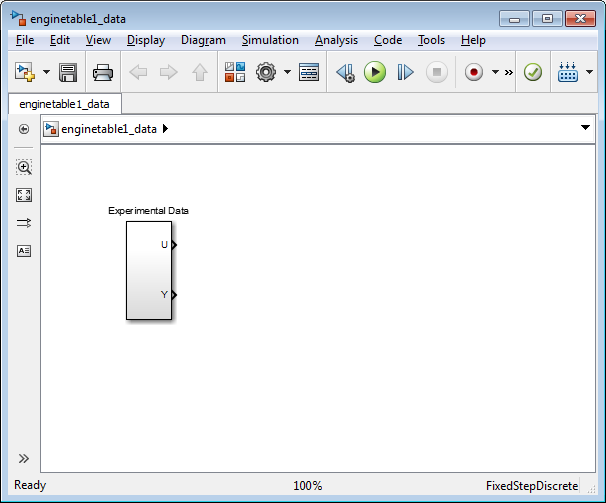This command also loads the variables `X`, `Y` and `Z` into the MATLAB workspace. To learn more about this data, see About the Data.

1. Open the Simulink Library Browser.

At the MATLAB prompt, enter `slLibraryBrowser`.

2. Open the Simulink Design Optimization™ library.

In the Libraries pane, expand the Simulink Design Optimization node.

3. In the Simulink Design Optimization library tree, click Adaptive Lookup Tables.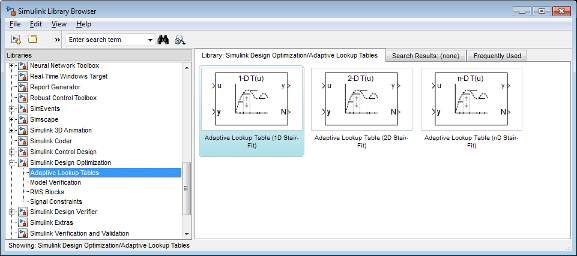4. Drag and drop the Adaptive Lookup Table (nD Stair-Fit) block from the Adaptive Lookup Tables library to the Simulink model window.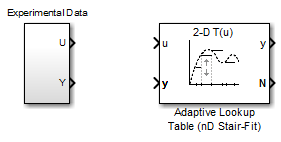3. Double-click the Adaptive Lookup Table (nD Stair-Fit) block to open the Function Block Parameters: Adaptive Lookup Table (nD Stair-Fit) dialog box.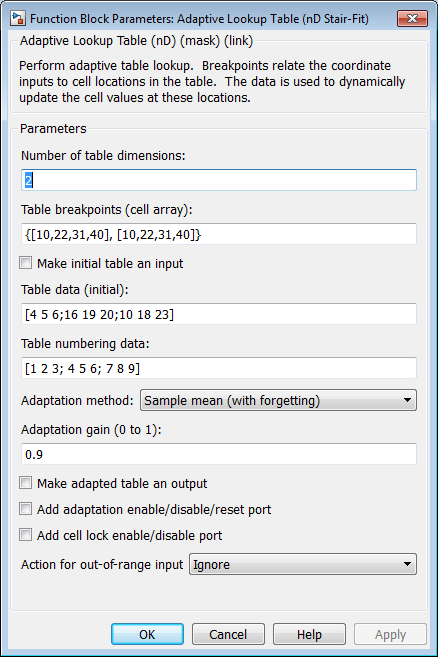4. In the Function Block Parameters dialog box:

1. Specify the following block parameters:

• Table breakpoints (cell array) — Enter ```{[X; 110], [Y; 7200]}``` to specify the range of input breakpoints.

• Table data (initial) — Enter `rand(10,36)` to specify random numbers as the initial table values for the volumetric efficiency.

• Table numbering data — Enter `reshape(1:360,10,36)` to specify a numbering scheme for the table cells.

2. Verify that `Sample mean (with forgetting)` is selected in the Adaptation method drop-down list.

3. Enter `0.98` in the Adaptation gain (0 to 1) field to specify the forgetting factor for the ```Sample mean (with forgetting)``` adaptation algorithm.

An adaptation gain close to 1 indicates high robustness of the lookup table values to input noise. To learn more about the adaptation gain, see Sample Mean with Forgetting in Selecting an Adaptation Method.

4. Select the Make adapted table an output check box.

This action adds a new port named `Tout` to the Adaptive Lookup Table block. You use this port to plot the table values as they are being adapted.

This action adds a new port named `Enable` to the Adaptive Lookup Table block. You use this port to enable or disable the adaptation process.

6. Select the Add cell lock enable/disable port check box.

This action adds a new port named `Lock` to the Adaptive Lookup Table block. You use this port to lock a cell during the adaptation process.

7. Verify that `Ignore` is selected in the Action for out-of-range drop-down list.

This selection specifies that the software ignores any time-varying inputs outside the range of input breakpoints during adaptation.

Tip

After you configure the parameters, the block parameters dialog box looks like the following figure.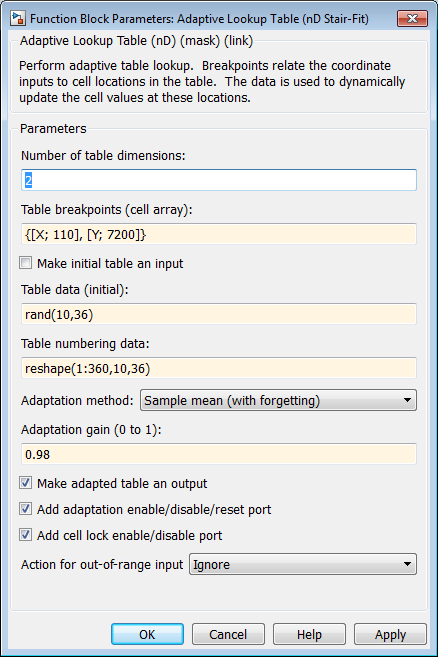8. Click to close the Function Block Parameters dialog box.

The Simulink model now looks similar to the following figure.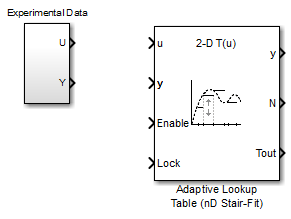5. Assign the input and output data to the engine model by connecting the `U` and `Y` ports of the Experimental Data block to the `u` and `y` ports of the Adaptive Lookup Table block, respectively.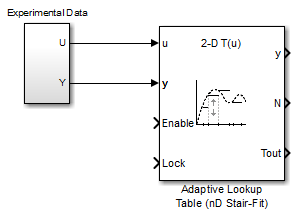6. Design a logic using Simulink blocks to enable or disable the adaptation process. Connect the logic to the Adaptive Lookup Table block, as shown in the following figure.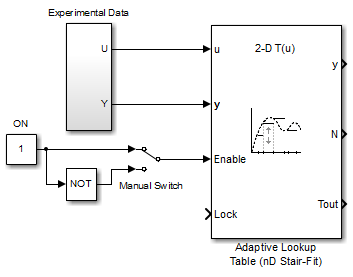This logic outputs an initial value of 1 which enables the adaptation process.

7. Design a logic to lock a cell during adaptation. Connect the logic to the Adaptive Lookup Table block, as shown in the following figure.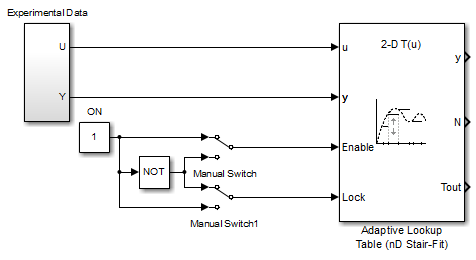8. In the Simulink Library Browser, select the Simulink > Sinks library, and drag Display blocks to the model window. Connect the blocks, as shown in the following figure.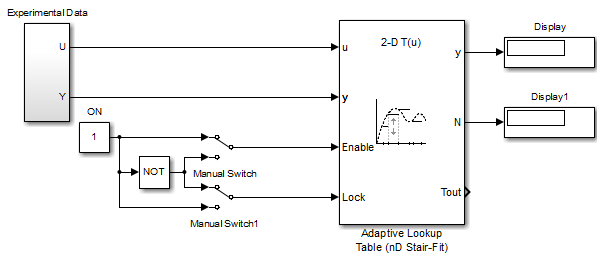During simulation, the Display blocks show the following:

• `Display` block — Shows the value of the current cell being adapted.

• `Display1` block — Shows the number of the current cell being adapted.

9. Write a MATLAB function to plot the lookup table values as they adapt during simulation.

Alternatively, type `enginetable` at the MATLAB prompt to open a preconfigured Simulink model. The `Efficiency Surface` subsystem contains a function to plot the lookup table values, as shown in the next figure.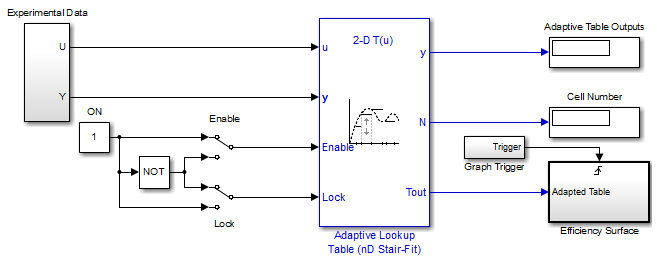10. Connect a To Workspace block to export the adapted table values:

1. In the Simulink Library Browser, select the Simulink > Sinks library, and drag the To Workspace block to the model window.

2. Double-click the To Workspace block to open the Sink Block Parameters dialog box, and type `Tout` in the Variable name field.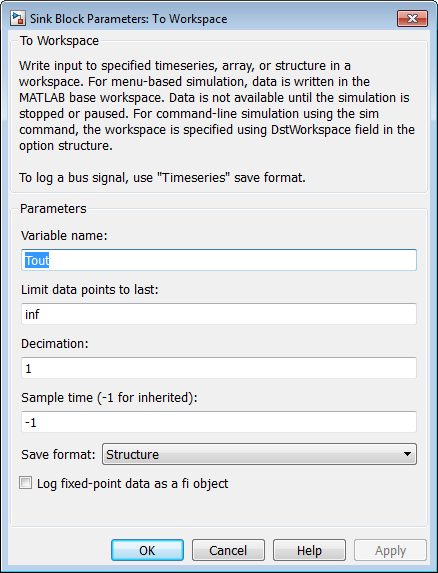3. Click .

4. Connect the To Workspace block to the adaptive lookup table output signal `Tout`, as shown in the next figure.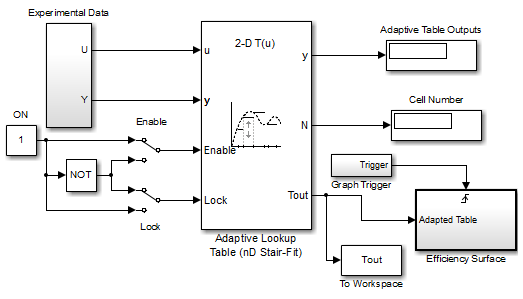You have now built the model for updating and viewing the adaptive lookup table values. You must now simulate the model to start the adaptation, as described in Adapting the Lookup Table Values Using Time-Varying I/O Data.

### Adapting the Lookup Table Values Using Time-Varying I/O Data

In this portion of the tutorial, you learn how to update the lookup table values to adapt to the time-varying input and output values.

You must have already built the Simulink model, as described Building a Model Using Adaptive Lookup Table Blocks.

1. In the Simulink Editor, specify the simulation time as `inf`.

The simulation time of infinity specifies that the adaptation process continues as long as the input and output values of the engine change.

2. In the Simulink Editor, click Run under Simulation to start the adaptation process.

A figure window opens that shows the volumetric efficiency of the engine as a function of the intake manifold pressure and engine speed:

• The left plot shows the measured volumetric efficiency as a function of intake manifold pressure and engine speed.

• The right plot shows the volumetric efficiency as it adapts with the time-varying intake manifold pressure and engine speed.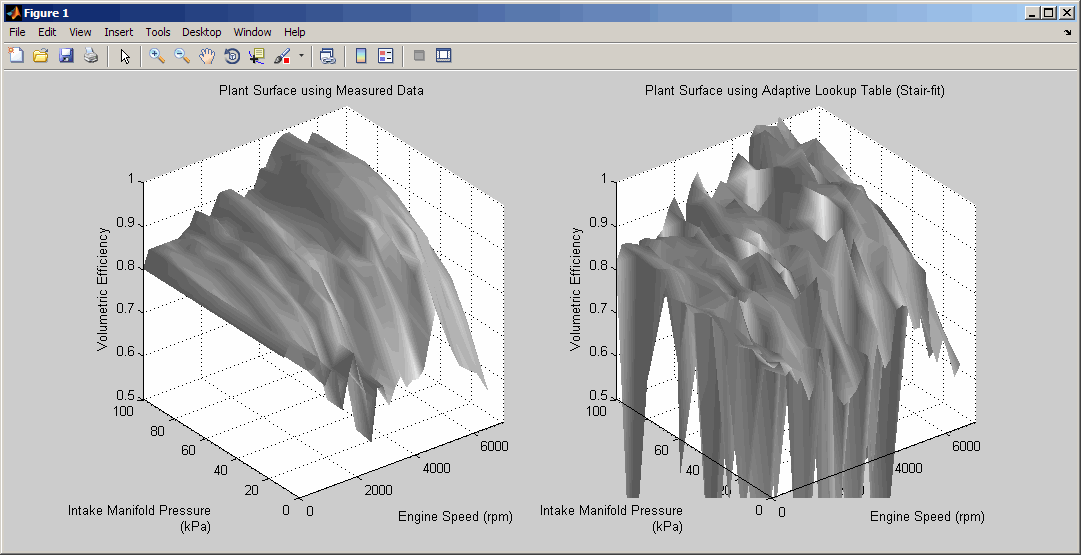During simulation, the lookup table values displayed on the right plot adapt to the variations in the I/O data. The left and the right plots resemble each other after a few seconds, as shown in the next figure.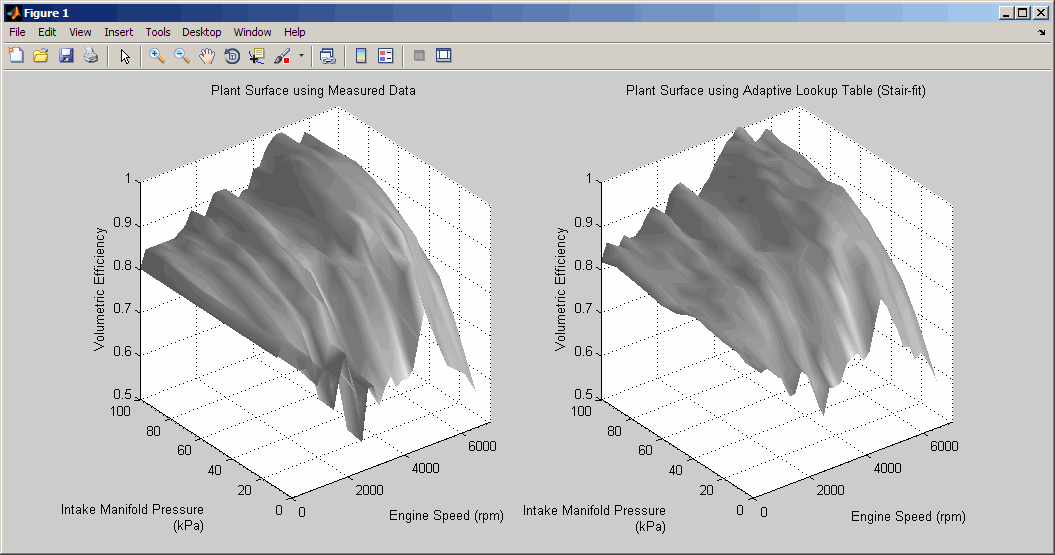Tip

During simulation, the `Cell Number` and ```Adaptive Table Outputs``` blocks in the Simulink model display the cell number, and the adapted lookup table value in the cell, respectively.

3. Pause the simulation by clicking Pause under Simulation.

This action also exports the adapted table values `Tout` to the MATLAB workspace.

Note

After you pause the simulation, the adapted table values are stored in the Adaptive Lookup Table block.

4. Examine that the left and the right plots match. This resemblance indicates that the table values have adapted to the time-varying I/O data.

5. Lock a table cell so that only one cell adapts. You may find this feature useful if a portion of the data is highly erratic or otherwise difficult for the algorithm to handle.

1. Click Run under Simulation to restart the simulation.

2. Double-click the `Lock` block. This action toggles the switch and feeds the output of the `ON` block to the`Lock` input port of the `Adaptive Lookup Table(nD Stair-Fit)` block.

You can view the number of the locked cell in the `Cell Number` block in the Simulink model.

6. After the table values adapt to the time-varying I/O data, you can continue to use the Adaptive Lookup Table block as a static lookup table:

1. In the Simulink model window, double-click the `Enable` block. This action toggles the switch, and disables the adaptation.

2. Click Run under Simulation to restart the simulation, if it is not already running.

During simulation, the Adaptive Lookup Table block works like a static lookup table, and continues to estimate the output values as the input values change. You can see the current lookup table value in the `Adaptive Table Outputs` block in the Simulink model window.

Note

After you disable the adaptation, the Adaptive Lookup Table block does not update the stored table values, and the figure that displays the table values does not update.